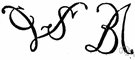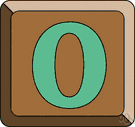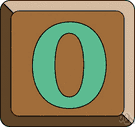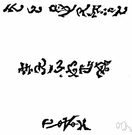cipher

(redirected from cyphered)
Also found in: Thesaurus, Legal, Encyclopedia.
Related to cyphered: ciphered

ci·pher

also cy·pher (sī′fər)
n.
1. The mathematical symbol (0) denoting absence of quantity; zero.
2. An Arabic numeral or figure; a number.
3. One having no influence or value; a nonentity.
4.
a. A cryptographic system in which units of text of regular length, usually letters, are transposed or substituted according to a predetermined code.
b. The key to such a system.
c. A message written or transmitted in such a system.
5. A design combining or interweaving letters or initials; a monogram.
v. ci·phered, ci·pher·ing, ci·phers also cy·phered or cy·pher·ing or cy·phered
v.intr.
To solve problems in arithmetic; calculate.
v.tr.
1. To put in secret writing; encode.
2. To solve by means of arithmetic.

[Middle English cifre, from Old French, from Medieval Latin cifra, from Arabic ṣifr, from ṣafira, to be empty (translation of Sanskrit śūnyam, cipher, dot); see ṣpr in Semitic roots.]

(ˈsaɪfə) or

cypher

n
1. a method of secret writing using substitution or transposition of letters according to a key
2. a secret message
3. the key to a secret message
4. (Mathematics) an obsolete name for zero1
5. (Mathematics) any of the Arabic numerals (0, 1, 2, 3, etc, to 9) or the Arabic system of numbering as a whole
6. a person or thing of no importance; nonentity
7. a design consisting of interwoven letters; monogram
8. (Instruments) music a defect in an organ resulting in the continuous sounding of a pipe, the key of which has not been depressed
vb
9. to put (a message) into secret writing
10. (Instruments) (intr) (of an organ pipe) to sound without having the appropriate key depressed
11. (Mathematics) rare to perform (a calculation) arithmetically
[C14: from Old French cifre zero, from Medieval Latin cifra, from Arabic sifr zero, empty]

ci•pher

(ˈsaɪ fər)

n.
1. zero.
2. any of the Arabic numerals or figures.
3. a person or thing of no value or importance; nonentity.
4.
a. a secret method of writing, as by code.
b. writing done by such a method; a coded message.
5. the key to a secret method of writing.
6. a combination of letters, as the initials of a name; monogram.
v.i.
7. to use figures or numerals arithmetically.
8. to write in or as in cipher.
v.t.
9. to calculate numerically; figure.
10. to convert into cipher; encipher.
Also, esp. Brit., cypher.
[1350–1400; Middle English siphre < Medieval Latin ciphra < Arabic ṣifr empty, zero; translation of Skt śūnyā empty]
ci′pher•er, n.
zero, cipher - Zero and cipher come from Arabic sifr, "nought, zero," from Sanskrit sunya, "empty."

cipher

Any cryptographic system in which arbitrary symbols (or groups of symbols) represent units of plain text of regular length, usually single letters; units of plain text are rearranged; or both, in accordance with certain predetermined rules. See also cryptosystem.

cipher

Past participle: ciphered
Gerund: ciphering

Imperative
cipher
cipher
Present
I cipher
you cipher
he/she/it ciphers
we cipher
you cipher
they cipher
Preterite
I ciphered
you ciphered
he/she/it ciphered
we ciphered
you ciphered
they ciphered
Present Continuous
I am ciphering
you are ciphering
he/she/it is ciphering
we are ciphering
you are ciphering
they are ciphering
Present Perfect
I have ciphered
you have ciphered
he/she/it has ciphered
we have ciphered
you have ciphered
they have ciphered
Past Continuous
I was ciphering
you were ciphering
he/she/it was ciphering
we were ciphering
you were ciphering
they were ciphering
Past Perfect
Future
I will cipher
you will cipher
he/she/it will cipher
we will cipher
you will cipher
they will cipher
Future Perfect
I will have ciphered
you will have ciphered
he/she/it will have ciphered
we will have ciphered
you will have ciphered
they will have ciphered
Future Continuous
I will be ciphering
you will be ciphering
he/she/it will be ciphering
we will be ciphering
you will be ciphering
they will be ciphering
Present Perfect Continuous
I have been ciphering
you have been ciphering
he/she/it has been ciphering
we have been ciphering
you have been ciphering
they have been ciphering
Future Perfect Continuous
I will have been ciphering
you will have been ciphering
he/she/it will have been ciphering
we will have been ciphering
you will have been ciphering
they will have been ciphering
Past Perfect Continuous
I had been ciphering
you had been ciphering
he/she/it had been ciphering
we had been ciphering
you had been ciphering
they had been ciphering
Conditional
I would cipher
you would cipher
he/she/it would cipher
we would cipher
you would cipher
they would cipher
Past Conditional
I would have ciphered
you would have ciphered
he/she/it would have ciphered
we would have ciphered
you would have ciphered
they would have ciphered
ThesaurusAntonymsRelated WordsSynonymsLegend:
 Noun 1cipher - a message written in a secret code  cyphermessage - a communication (usually brief) that is written or spoken or signaled; "he sent a three-word message" 2cipher - a mathematical element that when added to another number yields the same numberdigit, figure - one of the elements that collectively form a system of numeration; "0 and 1 are digits" 3cipher - a quantity of no importance; "it looked like nothing I had ever seen before"; "reduced to nil all the work we had done"; "we racked up a pathetic goose egg"; "it was all for naught"; "I didn't hear zilch about it"relative quantity - a quantity relative to some purposenihil - (Latin) nil; nothing (as used by a sheriff after an unsuccessful effort to serve a writ); "nihil habet" 4 cipher - a person of no influencecommon man, common person, commoner - a person who holds no titlepip-squeak, small fry, squirt - someone who is small and insignificantjackanapes, whippersnapper, lightweight - someone who is unimportant but cheeky and presumptuous 5cipher - a secret method of writing    code - a coding system used for transmitting messages requiring brevity or secrecy Verb 1 cipher - convert ordinary language into code; "We should encode the message for security reasons"encode - convert information into code; "encode pictures digitally" 2 cipher - make a mathematical calculation or computationmath, mathematics, maths - a science (or group of related sciences) dealing with the logic of quantity and shape and arrangementreason - think logically; "The children must learn to reason"quantise, quantize - apply quantum theory to; restrict the number of possible values of (a quantity) or states of (a physical entity or system) so that certain variables can assume only certain discrete magnitudes that are integral multiples of a common factor; "Quantize gravity"work out - be calculated; "The fees work out to less than \$1,000"extract - calculate the root of a numberprocess - perform mathematical and logical operations on (data) according to programmed instructions in order to obtain the required information; "The results of the elections were still being processed when he gave his acceptance speech"prorate - divide or assess proportionally; "The rent was prorated for the rest of the month"miscalculate, misestimate - calculate incorrectly; "I miscalculated the number of guests at the wedding"recalculate - calculate anew; "The costs had to be recalculated"average out, average - compute the average offactor, factor in, factor out - resolve into factors; "a quantum computer can factor the number 15"add together, add - make an addition by combining numbers; "Add 27 and 49, please!"deduct, subtract, take off - make a subtraction; "subtract this amount from my paycheck"multiply - combine by multiplication; "multiply 10 by 15"fraction, divide - perform a division; "Can you divide 49 by seven?"interpolate, extrapolate - estimate the value ofdifferentiate - calculate a derivative; take the derivativeintegrate - calculate the integral of; calculate by integrationsurvey - plot a map of (land)estimate, gauge, approximate, guess, judge - judge tentatively or form an estimate of (quantities or time); "I estimate this chicken to weigh three pounds"budget - make a budgetcapitalise, capitalize - compute the present value of a business or an incomesolve, resolve - find the solution; "solve an equation"; "solve for x"

cipher

noun
1. code, coded message, cryptogram The codebreakers cracked the cipher.
2. They were little more than ciphers who faithfully carried out the Fuehrer's commands.

cipher

noun
A totally insignificant person:
Informal: pip-squeak, zero.
Slang: shrimp, zilch.
verb
To ascertain by mathematics:
Translations
شِفْرَه، رُموز
šifracifračíslicekód
kodekodeskrift
numerosalakirjoitussalakirjoitusjärjestelmäsalakirjoitusviesti
titkosírászérónulla
dulmál
šifras
kodsšifrs
šifrované písmo
chiffersiffra

cipher

[ˈsaɪfəʳ]
A. N
1. (= 0, zero) → cero m; (= any number, initials) → ; (= Arabic numeral) → cifra f, número m
2. (= secret writing) → cifra f, código m
in ciphercifrado, en clave
3. (= monogram) →
4. (fig) (= person) he's a mere cipheres un cero a la izquierda
B. VT
1. [+ code, calculations, communications] → cifrar
2. (Math) → calcular

cipher

[ˈsaɪfər] n
(= code) →
in cipher →
(= faceless employee) →

cipher

n
(= Arabic numeral)Ziffer f, → Zahl f
(= zero)Null f
(= nonentity)Niemand m no pl
(= code)Chiffre f, → Code m, → Kode m; in cipherchiffriert
(= monogram)Monogramm nt, → Namenszeichen nt
vt (= encode)

cipher

[ˈsaɪfəʳ] n (code) → codice m (cifrato) (Math) → zero (fig) (faceless employee) → persona di nessun conto, nullità f inv
in cipher → in codice

cipher

(ˈsaifə) noun
secret writing; a code. The message was written in cipher.
Site: Follow: Share:
Open / Close